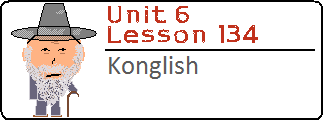Lesson 134: This lesson is an organization of all of the English-based words I could come up with. I talk about these “Konglish” words in three sections: words borrowed from English where the meaning is different than the English usage; words that are a combination of English and Korean; words in Korean that have been directly borrowed from English.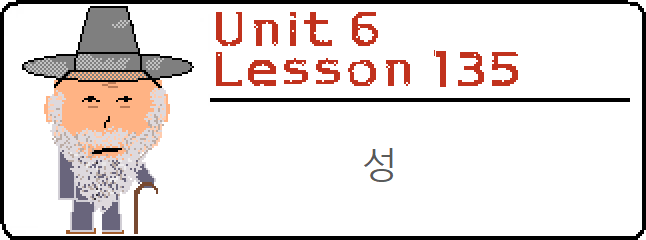Lesson 135: In this lesson, you will learn about the syllable 성. You will learn that 성 can have the meaning of a) Character or a range of some quality, b) completion, or c) loyalty and devotion.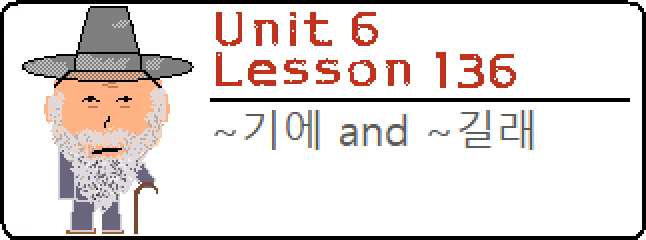Lesson 136: In this lesson, you will learn about ~기에 and ~길래. These grammatical principles are used to create a meaning of “so” or “because,” which often leads learners of Korean confused with how these may be different than ~아/어서.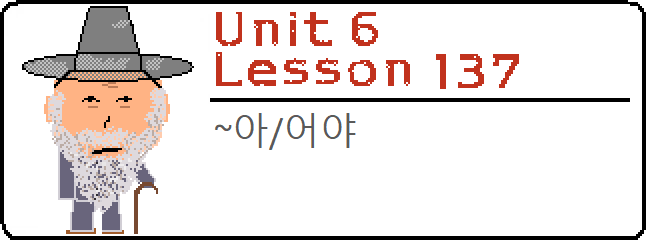Lesson 137: In this lesson, you will learn how to add ~아/어야 to the end of a clause. You saw this grammatical principle in a previous lesson, but only followed by 하다. We will look at how this is used in all other situations.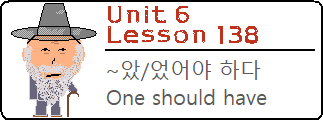Lesson 138: In this lesson, we will add complexity to a very common grammatical principle. ~아/어야 하다 was introduced in Lesson 46 as a way to indicate that one must do an action. In the previous lesson, I broke that grammatical principle down by explaining the purpose of ~아/어야 and even indicated that it can be used without the concluding 하다. In this lesson, you will see even more ways that ~아/어야 can be used. It is also possible to add ~아/어야 to a clause that already has the past tense marker ~았/었 in it. In this lesson, we will look at how you can use ~았/었어야 하다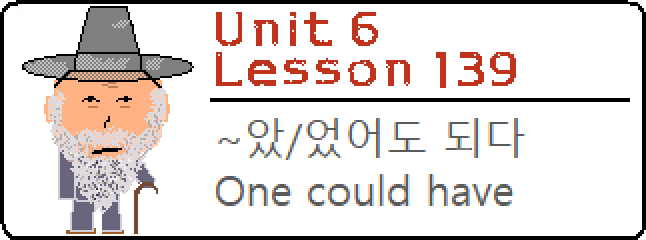Lesson 139: In this lesson, we will add complexity to a very common grammatical principle. ~아/어도 되다 was introduced in Lesson 49 as a way to indicate that one may do an action. Two lessons ago, I broke down the purpose of ~아/어야. In the previous lesson, I introduced the idea of including ~았/었 before ~아/어야. In this lesson, you will expand on these ideas to create sentences that end in ~았/었어도 되다. Let’s get started.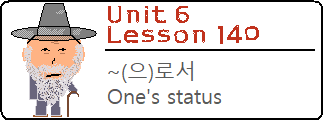Lesson 140: Two similar looking grammatical principles, two completely different meanings. In this lesson and the next one, you will learn how to use ~(으)로서 and ~(으)로써 in Korean sentences. Korean people often confuse which one is which because of their similar looking structures. However, they are completely different and with these two lessons it shouldn’t be hard for you to distinguish them.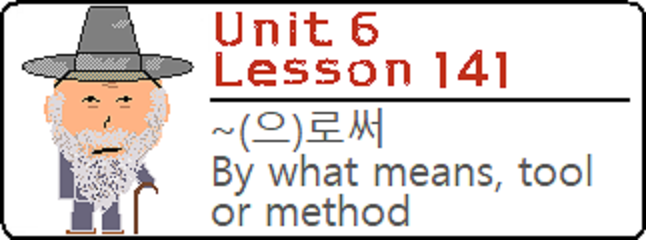Lesson 141: In this lesson, we will learn about ~(으)로써, which looks slightly similar to the grammatical principle ~(으)로서 from the previous lesson. Korean people often have trouble remembering which one is which, but let’s make sure that doesn’t happen to you!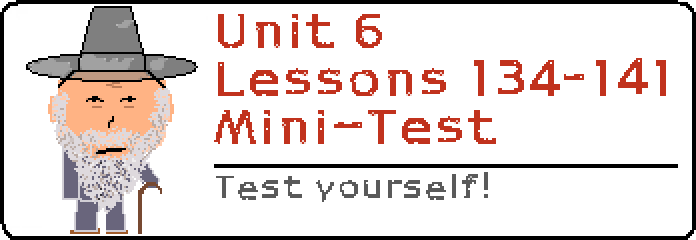Lessons 134 – 141 Mini Test: Test yourself on what you learned from Lessons 134 to 141! A Mini-Test like this will be uploaded after every eight lessons. Don’t worry if the test doesn’t go so well – this website is here to help you!